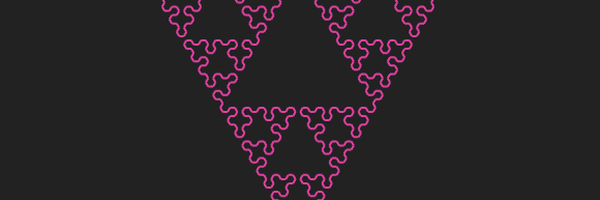# More fractals using rewriting systems

I've been working through a great book called The Algorithmic Beauty of Plants.

The first chapter introduces the concept of L-systems, where complex shapes can be built up by starting with an initial shape, and replacing each part of it according to a set of rules.

These simple shapes can be created using Turtle graphics: we start with a state defined by a position and an angle, and then we can either tell the turtle to:

• Move forward
• Turn left, or
• Turn right

As the turtle moves it draws a line where it's been (it's sometimes useful to have the turtle move without drawing a line as well).

In the simple L-systems we'll cover here, we represent the commands we give the turtle using simple strings:

• `F`: move forward.
• `-`: turn right.
• `+`: turn left.

Then we can draw our initial shape using a string like `F-F-F-F`, which draws a square when the turn angle is 90°. Each `F` moves the same distance, and each `-/+` turns by the same amount.

To create fractals from using this system, we take each part of the initial string and replace it according to a set of rules, like:

``````{'F': 'F-F+F+FF-F-F+F'}
``````

i.e. replacing each forward movement of the turtle with a series of smaller segments (the size of the smaller segments relative to the original is important for getting the right result, something I felt the book didn't explain well). Then you can repeat these replacements multiple times, replacing the segments with smaller and smaller copies each time.

Below, I've implemented a simple Turtle system that can understand these simple strings of commands and replacements, and I've used it to generate a few different fractals (all using the same common code with a few different settings). You can set the depth, the number of times we run the replacements, to 0 to see the initial shape.

## Koch island:

• The initial shape is `F-F-F-F` (a square).
• The replacement rules are `{'F': 'F-F+F+FF-F-F+F'}`
• The turn angle is 90°
• The segment length decreases by a factor of 4 each time we replace
Depth:

## Koch curve:

• The initial shape is `F-F-F-F` (a square again)
• The replacement rules are `{'F': 'FF-F-F-F-F-F+F'}`
• The turn angle is 90°
• The segment length decreases by a factor of 3 each time
Depth:

This one requires a slight tweak to the rules: instead of just `F`, we have `L` and `R`: both are still just forward movements, but they can be replaced by different sequences to allow things to branch off in different directions.
• The initial shape is `R` (just a line)
• The replacement rules are: `{'L': 'R+L+R', 'R': 'L-R-L'}`